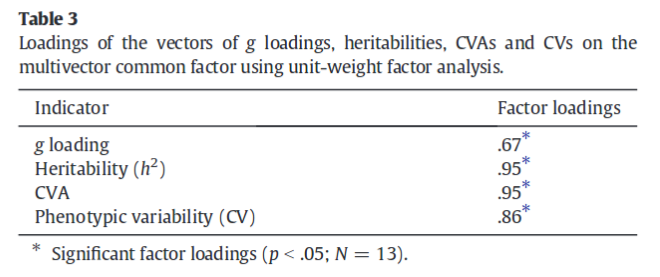Just a minor stats point for an otherwise excellent paper.

They use unit weighted factor analysis, which sounds fancy, but it is just averaging the z-scored versions of each indicator. What this does is assume that all variables load on a general factor, whereas ordinary exploratory factor analysis methods treat this as something to be tested. Thus, if one can make the assumption from theory or prior studies, one can dispense of this need to test, and thus gain some precision. From their paper, we have this table.Where they test the null hypothesis of r = .00. However, because UWFA is used, this is now incorrect because we do expect a positive correlation under the null hypothesis, which we can take as uncorrelated variables. The expected correlation based on chance is r = sqrt(1/k), where k is the number of indicators. This is because the composite variable gets its variance from each indicator evenly given r’s = 0 (or uniform) and the correlation is the square root of the variance contribution. Here’s an empirical demonstration assuming k = 4 which means that we expect r = sqrt(1/4) = sqrt(.25) = .50.

```MASS::mvrnorm(n = 10e3, mu = rep(0, 4), empirical = T, Sigma = matrix(c(1, 0, 0, 0, 0, 1, 0, 0, 0, 0, 1, 0, 0, 0, 0, 1), ncol = 4)) %>% as.data.frame() %>% set_colnames(letters[1:4]) %>% mutate(g = a+b+c+d) %>% cor() %>% round(2)
a b c d g
a 1.0 0.0 0.0 0.0 0.5
b 0.0 1.0 0.0 0.0 0.5
c 0.0 0.0 1.0 0.0 0.5
d 0.0 0.0 0.0 1.0 0.5
g 0.5 0.5 0.5 0.5 1.0```

So, one would have to devise a test to find loadings that deviate from .50, either alone or as a group. For the group, one can presumably use some kinda of modified ANOVA approach, and for test, one can probably modified the usual test difference between two correlations formula. Or be lazy, and type in a really large sample size, like I did (using this):So, the p < .05 for this case is at .79 (one-tailed; .825 for two-tailed), meaning that 3 of the 4 correlations reported in Woodley et al are still p <.05.